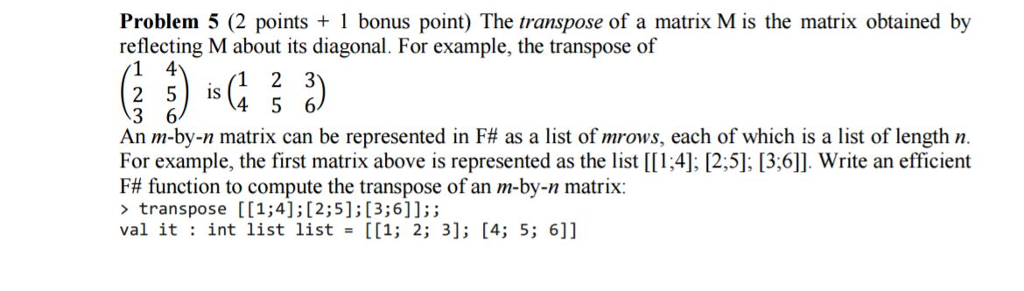# Answered! The transpose of a matrix M is the matrix obtained by reflecting M about its diagonal. For example, the transpose of…The transpose of a matrix M is the matrix obtained by reflecting M about its diagonal. For example, the transpose of (1 4 2 5 3 6) is (1 2 3 4 5 6) An m-by-n matrix can be represented in F# as a list of m rows, each of which is a list of length n. For example, the first matrix above is represented as the list [[1;4]:[2;5]:[3;6]]. Write an efficient F# function to compute the transpose of an m-by-n matrix: > transpose [[1;4];[2;5];[3;6]];: val it:int list list = [[1:2:3]:[4:5:6]]

write an efficient F# function to compute the transpose of an m-by-n matrix

Explanation:

Don't use plagiarized sources. Get Your Custom Essay on
Answered! The transpose of a matrix M is the matrix obtained by reflecting M about its diagonal. For example, the transpose of…
GET AN ESSAY WRITTEN FOR YOU FROM AS LOW AS \$13/PAGE

( _ :: _ ) :: _ is a pattern matching on value of type list of lists of ints

Hii i write the F# function which u want and ran the below code by using your input mention in your problem.

input:

[[1; 4]; [2; 5]; [3; 6]]

open System

let rec transpose = function
| (_::_)::_ as M -> List.map List.head M :: transpose (List.map List.tail M)
| _ -> []

[[1; 4]; [2; 5]; [3; 6]] |> transpose |> printfn “%A”

input:

[[1; 4]; [2; 5]; [3; 6]]

output:

[[1; 2; 3]; [4; 5; 6]]